# Polygons Worksheet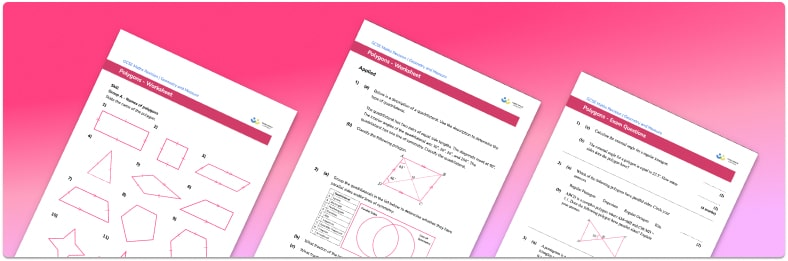• Section 1 of the polygons worksheet contains 36 skills-based polygons questions, in 3 groups to support differentiation
• Section 2 contains 4 applied polygons questions with a mix of worded problems and deeper problem solving questions
• Section 3 contains 4 foundation and higher level GCSE exam style polygons questions
• Answers and a mark scheme for all polygons questions are provided
• Questions follow variation theory with plenty of opportunities for students to work independently at their own level
• All questions created by fully qualified expert secondary maths teachers
• Suitable for GCSE maths revision for AQA, OCR and Edexcel exam boards

• This field is for validation purposes and should be left unchanged.

You can unsubscribe at any time (each email we send will contain an easy way to unsubscribe). To find out more about how we use your data, see our privacy policy.

### Polygons at a glance

Polygons are 2 dimensional shapes made from three or more straight lines. Some types of polygons, with their number of sides in brackets, are: all types of triangles (3), including equilateral triangles and isosceles triangles and all types of quadrilaterals (4), including trapezoids and rhombuses. Other polygons include pentagons (5), hexagons (6), heptagons (7), octagons (8), nonagons (9) and decagons (10).

There are regular and irregular polygons. Regular polygons are polygons where all of the sides are equal in length and all of the angles are the same whereas irregular polygons can have sides and angles of varying sizes. E.g. a regular hexagon has 6 equal sides and 6 equal interior angles.

For all polygons, the sum of the exterior angles is 360° and the sum of the interior angles is (n-2)*180° . Since the angles in regular polygons are all equal, the size of each interior angle of a regular polygon can be found by dividing the sum of the interior angles by the number of sides. For example, a regular pentagon has 5 sides and therefore the sum of the interior angles is (5-2)*180=540° , and each interior angle is 108° (540 divided by 5). In irregular polygons, knowing the sum of the interior angles for a polygon allows us to work out a missing angle within that polygon.

We can classify polygons using properties of polygons such as number of sides, any equal sides or angles and whether there are any parallel lines or lines of symmetry.

Looking forward, students can then progress to additional geometry worksheets, for example a 3D shapes worksheet or an area of a triangle worksheet.For more teaching and learning support on Geometry our GCSE maths lessons provide step by step support for all GCSE maths concepts.

## Related worksheets

Area Of 2D Shapes Worksheet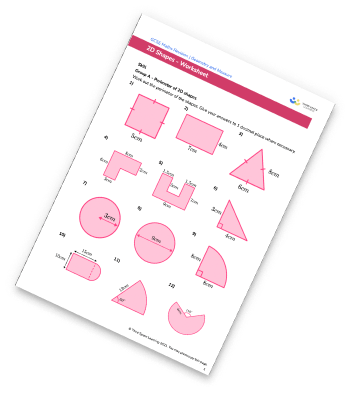Congruent Triangles Worksheet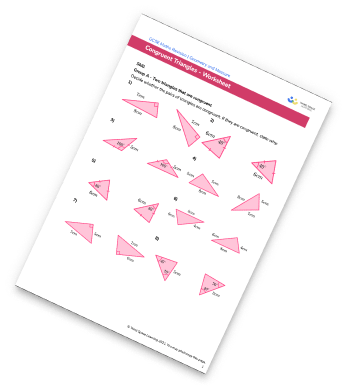Interior and Exterior Angles Worksheet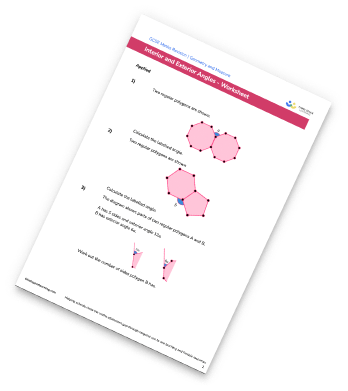Alternate Angles Worksheet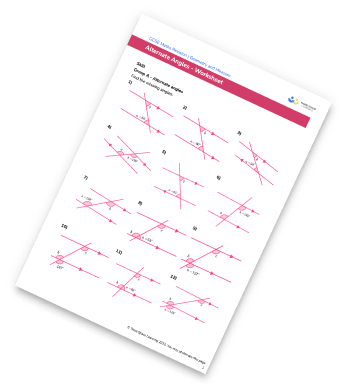## Do you have KS4 students who need more focused attention to succeed at GCSE?There will be students in your class who require individual attention to help them succeed in their maths GCSEs. In a class of 30, it’s not always easy to provide.

Help your students feel confident with exam-style questions and the strategies they’ll need to answer them correctly with our dedicated GCSE maths revision programme.

Lessons are selected to provide support where each student needs it most, and specially-trained GCSE maths tutors adapt the pitch and pace of each lesson. This ensures a personalised revision programme that raises grades and boosts confidence.

Find out more